# ColdFusion NumberFormat() Exploration

By on
Tags:

The other day, someone at the office asked me about adding parenthesis to his ColdFusion NumberFormat() function mask. I couldn't help him. I pretty much only ever use NumberFormat() to add mandatory decimal points and decimal places; in fact, I really use NumberFormat() to do what ColdFusion's DecimalFormat() function already does - I should really revaluate what I am doing in that case. Anyway, it occurred to me that most mask constructs of the NumberFormat() function are a total mystery to me. Time to do a little exploration.

One of the first things that I noticed in the ColdFusion NumberFormat() documentation is that if the value passed to NumberFormat() is a empty string, ColdFusion will convert it to 0. Therefore, if running this code:

``````<!---
If an empty string is passed to the NumberFormat()
function, ColdFusion converts it to a zero.
--->
#NumberFormat( "", "0.00" )#
``````

... we get the following output:

0.00

I am not really sure what the function of this feature is for? I could understand if NumberFormat() would convert any non-numeric string to zero, but it doesn't. If I tried to run this:

``````#NumberFormat( "ben", "0.00" )#
``````

... I would get the following ColdFusion error:

The value "ben" cannot be converted to a number.

So, it converts empty string, but I am probably never going to use that feature. That's probably more of a side effect of some internal process or something? I can't imagine they would have decided that empty string was OK as zero, but other strings were not.

That aside, what we really want to concentrate on are the available number masks. ColdFusion provides several special mask characters:

_ OR 9 - Digit placeholder - if there is a matching digit in the passed in number, it is displayed. Both _ and 9 do the same thing, only 9 shows decimal places a bit more clearly seen than the _ character. This mask item will not add any leading digits that are not in the given number - it will only display the leading digits that are already present. It will, however add trailing digits. Of course, if a digit is not present in the original value, it can only append zeros.

Running this code:

``````#NumberFormat( 4, "99.99" )#
``````

... gives us this output:

4.00

Notice that the 9 in the tens place did not add any leading zeros, but the 99 after the decimal place did add trailing zeros.

. - The period is the existing of or the location of a mandatory decimal point. This construct is used primarily in conjunction with other number masks. Used alone, it doesn't make a lot of sense. Running this code:

``````#NumberFormat( 4, "." )#
``````

... gives us this output:

Notice that the "." is now mandatory, but at the same time it doesn't make sense. In fact, I am not even sure if this is considered a valid number??? Really, the decimal place is meant to be used with something like the 0 mask of or the 9 mask. So, for instance, if we wanted to always show a number that had one decimal place after the zero, we could run this:

``````#NumberFormat( 3.16, ".9" )#
``````

This could give us the following output:

3.2

There's a few things to notice here. For starters, the 9 mask, when right of the decimal place will only show digits that match the mask. Any digits that appear right of the 9 number mask gets truncated. Also notice that when this truncation occurs, the number is rounded: .16 rounds up to be .2 in NumberFormat(). More importantly, realize that the 9 behaves in this way because of its placement in relation to the mandatory decimal place.

0 - The zero is a digit placeholder that does the same thing that the 9 mask does, only the zero mask will add leading zeros (unlike the 9 mask which will only add trailing zeros). Therefore, running this code:

``````#NumberFormat( 7.4, "000.000" )#
``````

... we get the following output:

007.400

Notice that not only where the 7 and 4 digits show, the 0 mask added two leading zero and two trailing zeros. This is the mask construct that I use most often to do exactly what decimal format does:

``````#NumberFormat( 7.4, ",.00" )#
``````

This forces the thousands separator, the mandatory decimal point, and two decimal places at all times. Like I said, I should really just be using ColdFusion's DecimalFormat() to do this.

( ) - This places parentheses around a number if that number is less than zero. Therefore, running this code:

``````#NumberFormat( -6.3, "()0.00" )#
``````

... gives us this output:

(6.30)

Notice that instead of using the minus sign, the 6.30 is placed in the parentheses. Also notice that our () mask does not surround the zeros in the number mask. This is not a requirement - NumberFormat() is just looking for mask "queues" and this one does NOT depend on placement (meaning, the placement of the () in the NumberFormat() mask should not ever change the output of the function). You can of course, use the parenthesis in a more readable format:

``````#NumberFormat( -6.3, "(0.00)" )#
``````

... but this is just a matter of personal preference; they both produce the same output.

If the number is a positive number then the function simply ignores the parentheses in the resultant output.

+ - The plus sign puts a plus sign before positive numbers and a minus sign before negative numbers. On it's own this is not so powerful, but when used in conjunction with other number masks, this will help to make sure that every value returned from ColdFusion's NumberFormat() is always the same length when either positive or negative in value. Running this code:

``````#NumberFormat( 5, "+" )#
``````

... we get the following output:

+5

And, running this code:

``````#NumberFormat( -5, "+" )#
``````

... we get the following output:

-5

Again, I think the real benefit of this is that we always get the same length value. Of course, if we had some sort of ledger system, the +/- would be a nice denoting of credit and debit.

- - Puts a space before positive numbers and a minus sign before negative numbers. Like the "+" number mask, this sort of mask allows us to produce values that are always the same length, whether positive or negative. Running this code:

``````[#NumberFormat( 8, "-" )#]
``````

... we get the following output:

[ 8]

Notice the leading space. And, just to drive home the point that NumberFormat() is looking for mask "queues", the minus sign or plus sign do NOT have to do at the beginning of your mask. For instance, running this code:

``````[#NumberFormat( 8, "0.00-" )#]
``````

... we get the following output:

[ 8.00]

Notice that after the 0.00 mask was applied, the "-" mask was properly applied even though it came after the mandatory decimal places. The position of the +/-, like the "()" mask construct, is not impacted by its placement and should be done so according to personal preference.

, - Separates every third decimal place with a comma (sometimes referred to as the thousands separator). Therefore, running the following code:

``````#NumberFormat( 123456789, "," )#
``````

... gives us the following output:

123,456,789

L,C,R - These position the value within in the number mask. L left justifies the value. C center justifies the value. R right justifies the value. These masks only have an affect when the digit place holder 9 (or .) is being used. If you use the 0 (zero) mask, these queues get ignored. In the following code, I am using ColdFusion's Replace() method to replace out spaces with * so that we can actually see how the replace works. Running this code:

``````#Replace( NumberFormat( 9, "L99999" ), " ", "*", "ALL" )#
#Replace( NumberFormat( 9, "C99999" ), " ", "*", "ALL" )#
#Replace( NumberFormat( 9, "R99999" ), " ", "*", "ALL" )#
``````

... we get the following output:

9****
**9**
****9

You can use this mask in conjunction with other masks, but things are a bit unpredictable.

\$ - Puts a dollar sign before formatted number. The document states that the first character of mask must be the dollar sign, bit in my experience, this is not true. Like many of the other masks used in ColdFusion's NumberFormat(), it is the existence of the \$ that is important, not its placement. Running this code:

``````#NumberFormat( 23, "\$" )#
``````

... gives us the following output:

\$23

Now, when using this in conjunction, things are little hairy at times. For instance, if we used this with a negative number:

``````#NumberFormat( -23, "\$" )#
``````

... we get:

-\$23

Notice that the minus sign comes before the \$ sign (not exactly what the documentation says it will do). However, if we add the parentheses mask:

``````#NumberFormat( -23, "(\$)" )#
``````

... we get the following output:

\$(23)

In this scenario, the dollar sign is outside of the parentheses even though in the previous example, the \$ sign was in between the "negative number notation" and the value. Not sure if that seems inconsistent or not, just take care when combining masks. Notice also that the placement of the \$, contrary to the documentation, is not important.

^ - This allows you to create different formatting for the left and right of the decimal place. To be honest, I could not figure out how this one works :)

Ok, so that gives me some more information about ColdFusion's NumberFormat(). Not a lot of surprises here, but I did learn some neat stuff that I might be able to apply at some point.

Want to use code from this post? Check out the license.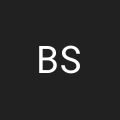One "feature" of NumberFormat that I have used is to convert ColdFusion boolean values to 1 or 0. This comes in handy when passing these values to SQL/JS/xml when you want a consistent format.

NumberFormat( "true" ) = 1

NumberFormat( "false" ) = 0

I have secretly fantasized that Val("true") would return 1... but alas it always returns 0 for any string.

Great summary.@Brett,

Val("true") might return 0, but (true * 1) will return 1.I'd imagine the #NumberFormat( "", "0.00" )# returning 0 is for when you are pulling data out of a query that has nulls. If it didn't you'd have to always test for "" since CF converts null values to empty strings.

Just my \$.02Nice... But... I'm still one step away though. What I am looking for is a single function call that will evaluate a loosely typed variable as a bit, while handling CFs three "yes,true,1" in the same way.

Val ( "true" * 1 ) works nicely, but Val ( "string" * 1 ) throws an error.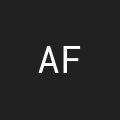Brett - I believe if you add YesNoFormat(), it should handle all the boolean options:

#NumberFormat(YesNoFormat("1"))# = 1
#NumberFormat(YesNoFormat("true"))# = 1
#NumberFormat(YesNoFormat("yes"))# = 1
#NumberFormat(YesNoFormat("0"))# = 0
#NumberFormat(YesNoFormat("false"))# = 0
#NumberFormat(YesNoFormat("no"))# = 0
#NumberFormat(YesNoFormat(""))# = 0 (it even works for blank)I've been using your method for a bit, thanks btw! But alas, I found the next problem in it.... (I have a huge rant about cf functions brewing...)

This throws an error (cf8 j2ee), so close!

<cfset myVal = "brett">

<cfif NumberFormat(YesNoFormat( myVal ) ) >

<cfdump var="yep">

<cfelse>

<cfdump var="nope">

</cfif>I guess this is the final statement - boy aint CF kinky!

NumberFormat( YesNoFormat( val ( myVar ) ) )

AND

NumberFormat( YesNoFormat( val ( "my valuel" ) ) )@Brett,

ColdFusion is usually pretty good about converting back and fourth between strings and numbers. For the YesNoFormat() and NumberFormat(), I would assume that it would auto convert "Yes" to "1". Is that where the error is coming in? Or is the error happening at YesNoFormat() itself?Suppose I have some calculated fields on a form that should only display the two digits past the decimal, but I want to store the 4 digits past the decimal. How can I achieve this?@Connor,

You just need to use the appropriate number format mask. Try this:

<cfset value = 12.3456 />

#timeFormat( value, "00.00" )#

This outputs: 12.35

As you can see, this not only limits the output to the given number of post-decimal "0" place holders, it also rounds the amount correctly.@Ben,
But that would change the value passed to the database via stored proc.
I have a form that has a value being shown that I want to limit to two decimal places being displayed. (It is calculated) Yet when they hit submit, I want to pass the value with up to 4 decimal places. Does this make sense or do I need more caffeine?@Connor,

Oh gotcha - sorry, I thought you were formatting on the ColdFusion side. If you want to format a number on the Javascript side, you need to use the toFixed() method:

Of course, you will have to keep the original value in a hidden form field or something for the eventual form submission.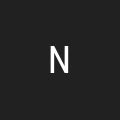I wanted to truncate decimal zeros where 20.123000 would be 20.123. So, I wrote a small function to loop over the reversed string and keep removing zeros...

Then I realized I could have just done numberWithoutTrailingZeros = numberWithTrailingZeros * 1;

Damn!I have set my db column to allow one place after the decimal. However, I do not want ColdFusion to display the decimal or the zero is the value is .0. Is this possible? I would like 5.0 to display as 5, but 5.5 to display at 5.5.@Jim,

If you simply want to truncate the value (without any rounding), you should be able to use the fix() method:

fix( 5.6 ) ==> 5

@Nikola,

Ahh, nice catch. Yeah, if you perform math, ColdFusion will convert it from a string to a number, which should get rid of the extraneous zeros.Ben - I have same question as Jim and I think maybe you misread it? I want numbers with non-zero decimal places to display the decimal, but those that have no decimal to display w/o the decimal point.

For example:

3.3 displays as 3.3
3.0 displays as 3

Fix will not do this. I cannot see how to do this with NumberFormat?@Andy,

To do that, you can bypass the numberFormat() approach and just get ColdFusion to see the values as "numbers." To do that, just multiply them by one:

<cfset x = 3.0 />
<cfset y = 3.3 />

x: #(x * 1)#<br />
y: #(y * 1)#

... when we run this, we get the following output:

x: 3
y: 3.3

By multiplying the values by 1, we are forcing ColdFusion to look at them as numbers (as opposed to strings that look like numbers). A that point, I believe ColdFusion wants to store them as the most simple numbers possible.

I don't know if this is a guaranteed approach. But it works for me.Thanks Ben - unfortunately that won't work for my situation. I was also using the "+" mask character. Basically this is for a for a football pool, so I need to list the point spreads as either "-6" or "+2.5" etc.

Of course, I can easily enough code some logic to do this, but the NumberFormat function seemed perfect.

Ah well...@Andy,

Ah, yeah, I think you'll need to do a little more manual logic to get that done.Ben - I have an additional question: can I use numberFormat() to round up to thousands - i.e. turn 123456 into 123,000 (and 987654 into 987,700)?

Greetings from Zurich, Switzerland.@Ralf,

Not that I know of. For that, you probably have to divide by 1000, round, and then multiple by 1000 and use number format.

``````#numberFormat(
ceiling( 987654 / 1000 ) * 1000
)#
``````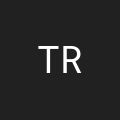Ben,

I too forgot that this existed. I've been pulling my hair out for days because my line chart wouldn't work. I came across this article and it hit me that I have null values in my query. Would have helped if I read something about this in the Charting documentation.

Tim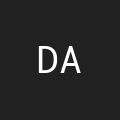Ben I want to format this currency from 450000.67 to 450,000.67, I have use Number Format Function but when I convert after point always disappear?
Can you give me how to format this currency.. ?
ThanksThanks for this posting. Although I did not see any posting for formatting for us currency with ZERO decimals I was able to figure that the this line does the trick:

NumberFormat(1000, "\$," )

This outputs: \$1,000

If there is a better way to do this please post away."Like I said, I should really just be using ColdFusion's DecimalFormat() to do this."

I believe (from my tests) DecimalFormat will not pad 0's though...disregard. It was the SerializeJSON bug stripping the trailing zeros. Had to surround result with quotes to force serializer to recognize the formatted number as a string.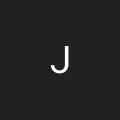Is there a way to mask a negative number?

Long story short in the finance sector when you go 'short' on a stock you want the price to fall this is a good thing because you are in profit.
I have a portfolio displaying current positions but I am stuck with profitable 'short' positions being displayed as -\$43.21 when they should really be \$43.21.

Any ideas?@Brett,

Good find, Brett. I just found this on my own as well. I am working on some migration utilities for a client and needed to convert "YES" and "NO" values to a SQL Server bit datatype. I'm using IsValid("boolean",value), and all that does is tell me if the value is "technically" valid. Using NumberFormat() after knowing the IsValid() check passed gives me a 1 or 0 -- exactly what I wanted.

AndyI have a client that keeps a cloth file with a factor stored in a column called "weight per running inch." This number is always less than 1 and stored with 6 digit decimal precision. Let's name this field "Weight"

Here's the problem. There seems to be no way to suppress leading zeros to the LEFT of the decimal point if the number is less than 1. For example, let's say Weight = .000507

#NumberFormat(Weight ,".000000")#
#NumberFormat(Weight ,"_.000000")#
#NumberFormat(Weight ,"9.000000")#

all produce 0.000507

Doesn't bother me, but my client wants to see .000507 not 0.000507

The only fix I've come up with is this:

#Mid(NumberFormat(Weight ,".000000"),2,7)#

This does produce .000507 but I find this coding rather confusing at first glance and inelegant -- even annoying! -- after closer study.

If anyone knows a better way to format this, I would love to hear it!